# Using Normal Force Measurements on a Rotational Rheometer to Evaluate Non-Linear Viscoelastic Effects

Viscoelasticity is the property of materials that have both viscous and elastic characteristics when undergoing deformation. When deformation occurs in viscoelastic materials, they undergo a three dimensional deformation that can be illustrated via a (3x3) tensor.

The tensor consists of three normal stresses, σxx, σ yy, and σ zz and the other six tensors constitute the shear stresses. If viscous behavior dominates, that is the fluid flows, then there would be just a single shear stress component, while the others can be overlooked. The initial normal stress variation can be desorbed as: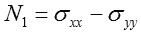Where σ yy is the stress that acts in the normal force direction and σ xx is the stress that acts in the direction of applied shear. In a rheological experiment, the normal force occurs in the axial direction and pertains to the upward thrust on the geometry and the bearing.

The normal stress variations are often shear rate dependent rather than shear stress dependent and hence can exhibit considerable increases with rising shear rate. Besides N1, the first normal stress coefficient can also be defined and this can be assumed as a viscoelastic equivalent to viscosity and depends on shear rate ý as per the equation given below: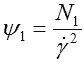Standard stress variations are related to non-linear effects and occur due to the core microstructure that becomes anisotropic under the flow conditions. Standard rheological effects are the 'die-swell' or 'post-extrusion swell' effect or the Weissenberg or 'rod-climbing' effect, and so on.

A wide range of products such as emulsions, surfactant systems, solutions, and polymer melts can have normal stresses. In a large number of instances, positive normal stresses exist; however, negative normal stresses also exist in certain cases, for instance in lamellar gels.

Cone and plate geometry is a perfect geometry that can be used for accurate measurement of the initial normal stress variation. This is because this type of geometry provides a consistent and reliable shear rate over the sample and upward thrust is only due to N1.

## Experimental Framework

In this analysis, the non-linear viscoelastic behavior of a body wash was initially assessed. A Kinexus rotational rheometer equipped with a cone-plate measuring system and a Peltier plate cartridge was used to make rotational rheometer measurements, by means of normal pre-configured sequences in the rSpace software.

Next, a standard loading sequence was utilized to make sure that both samples go through a controllable and reliable loading procedure. All rheology measurements were carried out at a temperature of 25°C. Utilizing an equilibrium table of shear rates test between 0.1 and 1000s-1, the flow curve was produced and the normal force was measured.

## Results and Discussion

The viscosity-shear rate curve for the body wash is shown in Figure 1. This body wash product is a shear thinning liquid as it exhibits Newtonian behavior at low shear rates followed by a sudden decrease in viscosity over a crucial shear rate.

Also, above this critical rate, a perceptible increase is seen in the normal force. This can be attributed to non-linear viscoelastic behavior induced by tension in the deforming microstructure.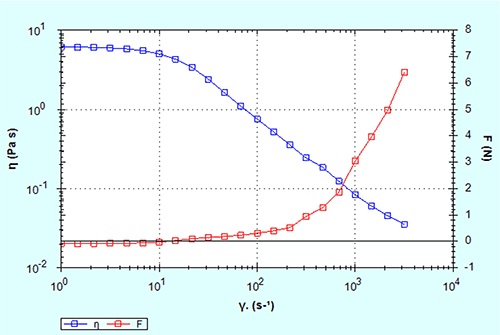Figure 1. Shear viscosity and normal force as a function of shear rate

This phenomenon is more perceptible when the normal stresses and shear stresses are directly compared (Figure 2). This demonstrates that the shear stress is exceeded by the normal stress at the point where the shear stress becomes constant.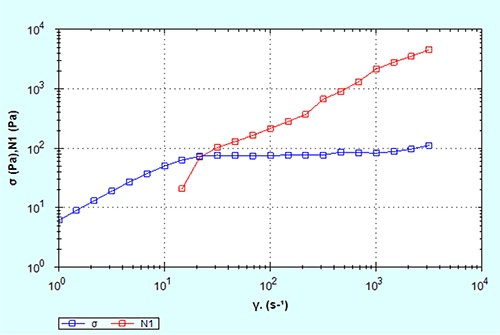Figure 2. Shear stress and normal stress as a function of shear rate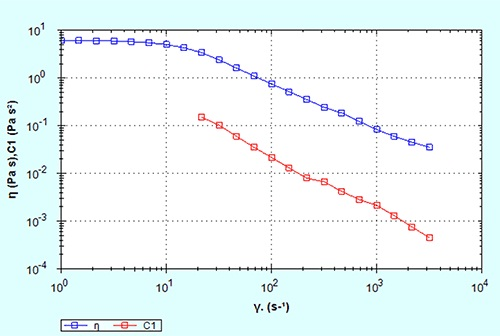Figure 3. Shows the first normal stress coefficient plotted alongside the shear

This matches with the elastic dominant flow behavior and elucidates why body washes structured by surfactants appear stringy and highly elastic when in use. This elastic dominant behavior will ultimately lead to instabilities in the flow behavior at high shear rates and the sample will scale out of the measurement gap.

The initial normal stress coefficient plotted alongside the shear viscosity is shown in Figure 3. Both coefficients exhibit analogous forms; however, since ψ1 is proportional to ý2 it is less than η in this situation and displays a steeper gradient.

In addition, comparing N1 or ψ1 and also viscosity for viscoelastic materials can prove useful, particularly if the material is highly viscoelastic and the type of application where the material is utilized is likely to possibly produce tension in the streamlines.

## Conclusion

In order to determine the non-linear viscoelastic behavior of a non-Newtonian material, the normal force should be measured as a virtue of shear rate by means of a cone-plate measuring system.

It is also possible to determine the initial normal stress coefficient and the initial normal stress variation that are equivalent to the shear viscosity and shear stress, respectively.This information has been sourced, reviewed and adapted from materials provided by Malvern Panalytical.

For more information on this source, please visit Malvern Panalytical.

## Citations

Please use one of the following formats to cite this article in your essay, paper or report:

• APA

Malvern Panalytical. (2019, September 03). Using Normal Force Measurements on a Rotational Rheometer to Evaluate Non-Linear Viscoelastic Effects. AZoM. Retrieved on September 15, 2019 from https://www.azom.com/article.aspx?ArticleID=12062.

• MLA

Malvern Panalytical. "Using Normal Force Measurements on a Rotational Rheometer to Evaluate Non-Linear Viscoelastic Effects". AZoM. 15 September 2019. <https://www.azom.com/article.aspx?ArticleID=12062>.

• Chicago

Malvern Panalytical. "Using Normal Force Measurements on a Rotational Rheometer to Evaluate Non-Linear Viscoelastic Effects". AZoM. https://www.azom.com/article.aspx?ArticleID=12062. (accessed September 15, 2019).

• Harvard

Malvern Panalytical. 2019. Using Normal Force Measurements on a Rotational Rheometer to Evaluate Non-Linear Viscoelastic Effects. AZoM, viewed 15 September 2019, https://www.azom.com/article.aspx?ArticleID=12062.

## Ask A Question

Do you have a question you'd like to ask regarding this article?